# Numerical Resolution of the Heat Equation in the Square Form Four-Part- II-

Numerical Resolution of the Heat Equation in the Square Form Four-Part- II-
Department of Physics, Faculty of Sciences, University Mohamed Boudiaf of M’sila, M’sila 28000, Algeria

Laboratory of Renewable Energy and Sustainable Development (LRESD), University Brothers Mentouri Constantinel, Constantinel 25000, Algeria

Laboratory of Energetic Physics, University Brothers Mentouri Constantine1, Constantinel 25000, Algeria

Laboratory of Electromechanical Systems (LASEM), National School of Engineers of Sfax (ENIS), University of Sfax, Sfax 3038, Tunisia

Corresponding Author Email:
djedid.taaloub@univ-msila.dz
Page:
205-211
|
DOI:
https://doi.org/10.18280/mmep.070206
21 May 2019
|
Accepted:
5 March 2020
|
Published:
30 June 2020
| Citation

OPEN ACCESS

Abstract:

This article is the second of a three-part document, which is to develop and validate the finite difference method applied to the thermal conduction, has a spatial variable and state with a heat source in a wall of a square shaped oven following a single direction, which results a differential equation partial of parabolic. The finite difference method is used to solve these equations numerically. The obtained schemes have been solved by the iterative method of GAUSS Seidel. This difference schemes have approximation order 0(τ+h2), and absolutely stable. The difference schemes are a system of linear algebraic equations whose solution can be found by the method of Gaussian elimination. View of the tri diagonal structure of this system can save the amount of calculation using the algorithm next sweep. The numerical results obtained prove the stability and the convergence of the schemes with differences and the computation algorithm elaborated.

Keywords:

iterative methods, numerical methods, recurrence formula, thermal conduction

1. Introduction

In very many sectors of the industry, the engineer and the technician are confronted with heat transfer problems that have been taken in recent years particularly important because of the high costs of energy and requirements of economies which result from it. Hence, the need to learn about the heat transfer. The transmission of heat (or heat transfer, or thermo kinetics, according to the authors) has become a very vast science, some of which date back to 1811 (FOURIER's law) or later, but which has undergone for some decades an intensive and productive study, to which the requirements of nuclear, solar and aerospace technologies as well as those resulting from the energy crisis [1-3]. Heat transfer is one of the most common modes of energy exchange. It intervenes naturally between two systems of which there exists between them a difference of temperature whatever the medium, even empty which separates them. As a result, heat transfer has a role that is often essential in pure science and technology applications. This role becomes even decisive when it is at the origin of the techniques used (heat exchangers, thermal engine, oven, lagging, use of solar energy, etc.…). The exact analytical solution of the heat equation when it exists can be very complex in the form of series containing eigenvalues often obtained by the numerical solution [4, 5]. The analytical solution may also not exist for different reasons, for example when the thermal conductivity varies with the temperature, or the case the boundary conditions are nonlinear, when the heat transfer coefficient is a function of the temperature or when there is a radiation heat exchange modeled by a nonlinear temperature polynomial [6-10]. If the analytical solution is impossible to obtain, it is necessary to seek another means to obtain an approximate solution, even in a certain number of points of the geometry only, the numerical methods are used to reach this goal. They are approximate mathematical methods to approximate either partial differential equations or integrals and transforming them into a system of easy-to-solve algebraic equations [11-14]. The study with numerical resolution of the heat conduction equation in a thick vertical wall submitted on one side to a uniform heat flux density, and to convective and radiative boundaries conditions on both sides. We determine the temperature-profile, the convective-radiative and conductive out puts on each face; versus the five dimensionless parameters concerned with that problem . A discretization of the PDEs by the three large families of methods: finite deference, finite volumes, and finite elements. It discretized the heat equation of monodimensional in a bar of 1 m in length and two-dimensional stationary in a rectangular field . The basic principles, which intervene in the heat transfer by pure conduction in permanent mode of the temperatures, are presented . The resolution the heat equation in the case of thin systems in a homogeneous isotropic immobile medium, with constant thermodynamic coefficients. It examined a simple case: the 1D slat subjected to thermal shock (without and with heat source volume) [18, 19]. The presentation the methods of approximation of solutions of partial differential equations (PDEs) by finite differences, while being basing on the particular case of the heat equation in dimension 1, then presenting the methods classics of numerical matrix analysis for the resolution of linear systems. The purpose is to motivate the use of these methods and to introduce the notations and basic properties used [20, 21]. The transfer the Black-Scholes equation to the heat equation that is easier to solve (numerically or analytically). Among the schemes presented, the most accurate and efficient way is to use a Crank -Nicholson method in time, and schemes with finite differences centered in space . The resolution numerically the cooling of the Moon in several cases. First, it will be satisfied to solve the heat equation in spherical coordinates in the case of a homogeneous material in order to become aware of the encountered difficulties. Then, it starts to complicate the thing with the introduction of a term of creation of heat, which is radioactivity. It will numerically solve the heat equation using different methods (finite differences and finite volumes) that will be compared in order to know their advantages and disadvantages. Finally, it will discuss the physical results obtained . The study numerical methods to solve the models commonly encountered in non-compressible fluid mechanics . The presentation a mathematical model for the characterization of thermal exchanges in variable regime between a flow of air in forced convection and a plane plate of finite thickness, whose back face is subjected to a constant temperature. The problem is treated by the method of KARMAN-POHLHAUSEN, unsteady case. The resolution of the integral energy equation is obtained by the fourth order Runge Kutta method . The presentation the principal modes of heat transfer: conduction, convection, and radiation and a chapter on heat exchangers. They showed that this course could solve concrete problems in many areas, for example: thermic of building, calculation of a solar collector, a heat exchanger . The treatment with the construction of random Crank-Nicolson solution for heat equation containing uncertainty through the coefficient. Under suitable hypotheses on data, we prove that the constructed random Crank-Nicolson solution is satisfying mean square convergent through the whole space .

This paper concentrates on solving the heat equation to a spatial variable using finite difference schemes of the second order approximation in spatial and temporal step 0 (h2 + τ). According to these difference schemes, the components of the solution at the fixed level can be calculated progressively from the components given at the initial level. These difference schemes represent a system of linear algebraic equations whose solution can be found by the GAUSS elimination method. Considering the tri diagonal structure of this system can save the calculation volume using the scanning algorithm. The solution is calculated according to the recurrence formula.

The aim of this paper is to develop and validate the finite difference method applied to thermal conduction, the case of a spatial and temporal variable with or without a heat source in a wall of the oven, and to know the distribution of temperature in a plane plate along an axis.

2. Method and Discretization

2.1 Concept of discretization

When concentrating on the temperature values at points of a grid, we replace the information contained in the differential equations by discrete values: the discretization. The algebraic equations of unknown Tij to mesh points that replaces the differential equations. To obtain these algebraic equations must make assumptions about changes in temperature between the points of the mesh and to express algebraically derived spatial and temporal temperature.

2.2 Structure of the discretization equation

The discretization equation is an algebraic relation linking the values of temperature for a number of mesh points. This is an equation derived from the differential equation (the mathematical model) and thus expresses the same physical information as the differential equation. When the number of grid points is large it is expected that the solution tends Green discretization the exact solution of the differential equation. For a given differential equation discretization of the equations are not unique but it is expected that all these equations give the same solution when the number of mesh points is great. The difference between the discrete equations is owed to the difference of the profiles chosen for the discretization.

3. Finite Difference Method

The finite differences method is the classical method of numerical solution of the partial derivative equations. It is the most suitable method because it allows an easier discretization of the equations, by replacing the derivative of the function by an approximation using finished differences; we obtain a linear system giving an approximation of the value of the function in several points. The discretization of the variables is obtained by dividing the studied domain by a grid of form and of dimensions adapted to each problem. Either in the plane (0, x, y) a domain D, be given on the domain a function to continue, tracing two families of lines: xi = i. Δx = i. h and yj = j. Δy = j. h or Δx, Δy are given numbers, i, j successively taking integer values.

## 1a.png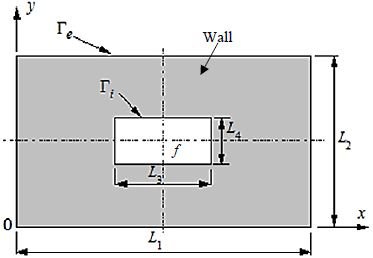(a)

## 1b.png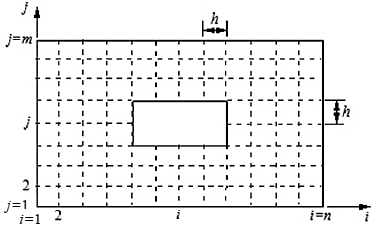(b)

Figure 1. (a): Geometry and (b): grid of the walls of the Oven

4. Description the Different Stages of Resolution by Finite Difference

We will consider the differential equation that governs the phenomenon of conduction in an oven (if the medium is homogeneous ρ, Κ and C are constants) which provided as follows.

$\frac{\partial T}{\partial t}=\alpha\left(\frac{\partial^{2} T}{\partial x^{2}}+\frac{\partial^{2} T}{\partial y^{2}}\right)+f$      (1)

With: $\alpha=K /(\rho . C)=\operatorname{cst}, \quad f=S /(\rho . C)$

α: is known "thermal diffusivity"

f: is thermal energy source,

To send completely the process of heat transfer should be given initial temperature distribution in the medium (initial condition) and thermal regime on the border (boundary condition).

We can consider different boundary conditions as follows:

1. The temperature T is given on Γ

T | Γ = T0

2. The heat flux on Γ is given: $k=\left.\frac{\partial T}{\partial n}\right|_{\Gamma}=T_{1}$

3. On the boundary Γ occur heat transfer by Newton's law:  $K \frac{\partial T}{\partial n}+\left.d\left(T-T_{0}\right)\right|_{\Gamma}=0$

K: heat transfer coefficient

T0: ambient temperature of the medium

Newton's law expresses the fact that the heat flux through the boundary is proportional al deviation of temperature on both sides of the border.

Note:

In the case of stationary temperature distribution does not depend on the time it has to say. Of the (1) we obtain the Poisson equation for the stationary distribution of heat.

$-\Delta T=\frac{f}{\alpha}$       (2)

If in the middle there is no heat source Poisson eq. (2) educe to Laplace equation

$\Delta T=0$     (3)

Eq. (3), it is the equation of the stationary distribution in the walls of an oven.

We use finite difference schemes for approximation of order two in spatial and time step 0(h2+τ) and absolutely stable (Appendix A).

5. The Problem of Heat Transfer to One Spatial Variables

The principle is exposed about one-dimensional problem, in the case of a wall with thermal source and constant or variable thermal characteristics, for which the analytical heat Eq. (1) is written:

$\left\{\begin{array}{c}\frac{\partial T}{\partial t}=\alpha\left(\frac{\partial^{2} T}{\partial x^{2}}\right)+f\left(x_{i}, t_{p+1}\right) \text { in } D=\{(x, t) \mid 0<x<1,0<t<{Ŧ}\} \\ T\left(x_{i}, 0\right)=\varphi\left(x_{i}\right) x \in[0,1], x_{i}=\text { ih intial condition } \\ T\left(0, t_{p}\right)=\Psi_{0}\left(t_{p}\right) t \in[0, {Ŧ}] \text { limit condition} \\ T\left(1, t_{p}\right)=\Psi_{1}\left(t_{p}\right) t \in[0, {Ŧ}] \text { limit condition } \\ 0 \leq t \leq {Ŧ}\end{array}\right.$      (4)

With the network Dh = {(xi, tp) = (i.h, p.τ) | i = 0... n; p = 0, 1... [Ŧ/ τ]}

The problem (4) approximated by differences in the schemes below:

$\left\{\begin{array}{c}\frac{T_{i}^{p+1}-T_{i}^{p}}{\tau}=\frac{\alpha}{h^{2}}\left(T_{i-1}^{p+1}-2 T_{i}^{p+1}+T_{i+1}^{p+1}\right)+f\left(x_{i}, t_{p+1}\right) \\ i=1, \ldots, n-1 ; p=0, \ldots,\left[\frac{{Ŧ}}{\tau}\right]-1 \\ T_{i}^{0}=\varphi\left(x_{i}\right) \quad ; \quad i=1, \ldots, n ; x_{i}=i . h ; h=1 / n \\ T_{0}^{p}=\psi_{0}\left(t_{p}\right), T_{n}^{p}=\psi_{1}\left(t_{p}\right) ; p=1, \ldots,\left[\frac{{Ŧ}}{\tau}\right] ; t_{p}=p . \tau\end{array}\right.$      (5)

This difference schemes have approximation order 0(τ+h2) and absolutely stable.

According to the difference schemes can be calculated progressively components of the solution at t = tp.

Tp ={Tpi , i=0,1,…,n }, p=1,2,…,[Ŧ/τ]    (6)

From components to the initial data

T0 ={T0i = φ(xi)    , i=0,1,…,n }    (7)

For each level t = tp fixed the difference schemes is a system of linear algebraic equations whose solution can be found by the method of Gaussian elimination.

View of the tri diagonal structure of this system can save the amount of calculation using the algorithm next sweep.

Either a tridiagonal system of linear algebraic equation:

$\left\{\begin{array}{l}a T_{i-1}^{\prime}+b T_{i}^{\prime}+a T_{i+1}^{\prime}=e_{i} \\ T_{0}^{\prime}=e_{0} ; \quad T_{n}^{\prime}=e_{n}\end{array}\right.$    (8)

The solution is calculated by the recurrence formula:

$\left\{\begin{array}{l}T_{n}^{\prime}=e_{n} \\ T_{i}^{\prime}=\alpha_{i} T_{i+1}^{\prime}+\beta_{i}, \quad i=n-1, \ldots, 0\end{array}\right.$    (9)

$T_{o}$ define the coefficients $\alpha_{i}, \beta_{i}$ we report:

$T_{i-1}^{\prime}=\alpha_{i-1} T_{i}^{\prime}+\beta_{i-1}$ in Eq. (9)

$a\left(\alpha_{i-1} T_{i}^{\prime}+\beta_{i-1}\right)+b T_{i}^{\prime}+a T_{i+1}^{\prime}=e_{i}$

Hence it takes:

$T_{i}^{\prime}=-\frac{a}{a \alpha_{i-1}+b} T_{i+1}^{\prime}+\frac{e_{i}-a \beta_{i-1}}{a \alpha_{i-1}+b}$     (10)

For (6) and (7) it follows:

$T_{0}^{\prime}=e_{0}$ and $T_{0}^{\prime}=\alpha_{0} T_{0}^{\prime}+\beta_{0}$ when $\alpha_{0}=0, \beta_{0}=e_{0}$

Comparing Eq. (10) and Eq. (9) we obtain the recurrence formula to determine the coefficients $\alpha_{i}$ and $\beta_{i}$:

$\left\{\begin{array}{c}\alpha_{0}=0, \quad \beta_{0}=e_{0} \\ \alpha_{i}=-\frac{a}{a \alpha_{i-1}+b}, \beta_{i}=\frac{e_{i}-a \beta_{i-1}}{a \alpha_{i-1}+b} ; i=1, \ldots, n-1\end{array}\right.$   (11)

So the scanning algorithm for solving tridiagonal system (9) consists of two steps:

Step 1: Calculate the coefficients $\alpha_{i}$ and $\beta_{i}$(i=0,…, n-1) according to Eq. (9)

Step 2: Calculate the components  $T_{i}^{\prime}$(i=n,…,0) according to Eq. (8)

Note

This problem is intended simultaneously to study the change of the temperature inside the furnace and in the walls with diffusivities different $\alpha=\alpha_{P}$ in the walls and $\alpha=$ $\alpha_{i}$ inside the furnace $\alpha_{P} \leq \alpha_{i}$ and $f=f_{P}=0$ in the walls.

6. Results and Discussion

Data:

$L=1, T=1, n=5, \tau=0.1, \alpha=0.1$

$f\left(x_{i}, t_{p+1 / 2}\right)=\sin \left(x_{i}\right) \cdot \operatorname{Exp}\left(-t_{p+1 / 2}\right) ; T\left(x_{i}, 0\right)=1$,

$T(0, t)=T(L, t)=0$

The Figures 2 and 3 show the isotherms for different values of sources and three-dimensional x, t and T if the problem of heat transfers to a variable spatial and temporal.

From the boundary conditions, the distribution of isothermal lines giving a very clear justification of the physical senses these conditions. Figures show the variation in temperature according to x and t for two different conditions S (heat source) where the source varying or zero. According to the figures we note the isotherms increases if S increases the hot zone and cold zone decreases, which implies a greater diffusion of heat generally. The temperature values belonging to S> 0 are higher than those corresponding to the case S = 0 then the greater the source more temperatures rise.

## 2.2a.png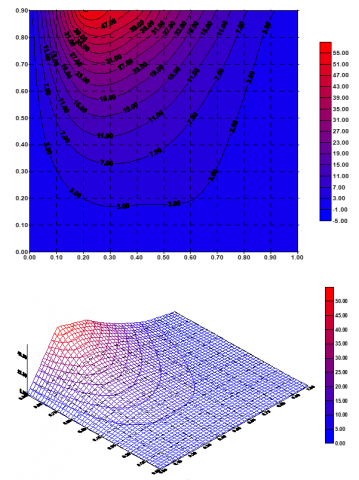(a)

## 2.2b.png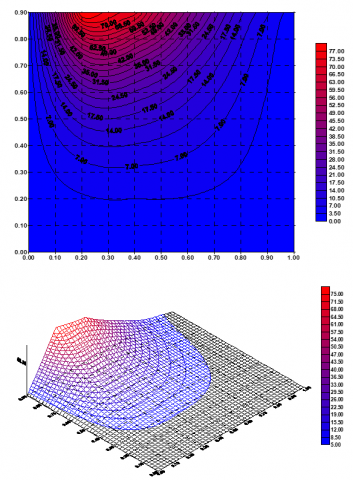(b)

Figure 2. Winding of isothermals (a) and (b) for TLt = T0t =0, without source

## 2.3a.png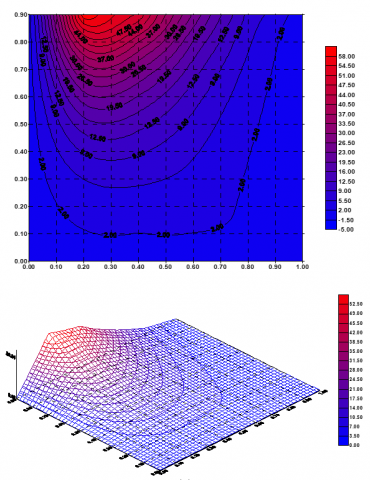(a)

## 2.3b.png(b)

Figure 3. Winding of isothermals (a) and (b) for TLt = T0t, =0, with source

7. Conclusion

In this paper we examined the problem solving method of heat transfer by conduction in a wall of an Oven following a single direction in the variable regime. The choice of method to adapt in this application can be guided by the following observations:

•   The numerical method is well suited to the problem whose geometry and boundary conditions are simple. It has the advantage of providing an exact solution, enabling rapid and accurate determination of the influence of various parameters.

•   If the geometry of the system and the boundary conditions are complex, then the numerical method is more appropriate.

The numerical results have complied with the physical phenomenon of the problem. Numerical results proved the stability and the convergence of difference schemes and algorithms developed. The difference schemes and the resolution algorithm presented in this article are applicable for any geometric configuration .

We can develop, generalize the problem study in this work in an optimal control problem in which we must choose the control parameter (the thermal source), to obtain the desired thermal regime, it is a problem very current in the design and operation of industrial oven.

Acknowledgment

The authors thank Lie Tien Pho for his help in implementing parts of the new algorithm used in the present study. The authors also thank Z. Driss for his help. We thank the reviewers for their rewarding comments on the present work; we are indebted to the editor for his stimulating encouragement.

Nomenclature
 a,b C Constants constant pressure heat D domain of resolution f thermal energy source, or function of x, y h k L p S t T T ' Tp X, xi Y, yj step according to x, y number of iteration length step time source of energy time temperature temperature wall temperature cartesian coordinates (i = 1,2,3) Cartesian coordinates (j = 1,2,3) Greek symbols Γ Ψ, φ wall surface function of x, y and t τ step time α, αp, αi αi ,βi thermal diffusivity, wall, internal coefficients ρ density Subscripts ∂ partial derivative d total derivative
APPENDIX A

About the approximation, the convergence and the stability of the difference schemes (5).

1. The order approximation 0(τ+h2)

It suffices to show that the norm of residual vector $\left\|\delta f^{h}\right\|_{F_{h}}$ is about 0(τ+h2).

In each interior node $\left(x_{i}, t_{p+1}\right)$ was:

$\left(\delta f^{h}\right)_{i}^{p+1}=\frac{T\left(x_{i}, t_{p+1}\right)-T\left(x_{i}, t_{p}\right)}{\tau}-\frac{\alpha}{h^{2}}\left(T\left(x_{i-1}, t_{p+1}\right)-\right.$$\left.2 T\left(x_{i}, t_{p+1}\right)+T\left(x_{i+1}, t_{p+1}\right)\right)-f\left(x_{i}, t_{p+1}\right) (12) Using the development formula of the node Taylor \left(x_{i}, t_{p+1}\right) was estimated: \left\{\begin{array}{c}\frac{T\left(x_{i}, t_{p+1}\right)-T\left(x_{i}, t_{p}\right)}{\tau}=\frac{\partial T}{\partial t}\left(x_{i}, t_{p+1}\right)+0(\tau) \\ \frac{1}{h^{2}}\left(T\left(x_{i-1}, t_{p+1}\right)-2 T\left(x_{i}, t_{p+1}\right)+T\left(x_{i+1}, t_{p+1}\right)\right)=\frac{\partial^{2} T}{\partial x^{2}}\left(x_{i}, t_{p+1}\right)+0\left(h^{2}\right)\end{array}\right. (13) Substituting Eq. (13) in Eq. (12) the estimate is derived: \left(\delta f^{h}\right)_{i}^{p+1}=\left(\frac{\partial T}{\partial t}-\alpha \frac{\partial^{2} T}{\partial x^{2}}-f\right)\left(x_{i}, t_{p+1}\right)+0\left(\tau+h^{2}\right) Since solving the problem of heat Eq. (4) Check: \frac{\partial T}{\partial t}-\alpha \frac{\partial^{2} T}{\partial x^{2}}-f=0 where, we have the estimate of the component of the residual vector at each node interior \left(\delta f^{h}\right)_{i}^{p+1}=0\left(\tau+h^{2}\right) Each node in the initial level t=0 or extremities x=0 or x=1 the approximation is exact that is to say: \left(\delta f^{h}\right)_{i}^{0}=\left(\delta f^{h}\right)_{0}^{p}=\left(\delta f^{h}\right)_{i}^{p}=0 where: \left\|\delta f^{h}\right\|_{F_{h}}=\max _{i, p}\left|\left(\delta f^{h}\right)_{i}^{p}\right|=0\left(\tau+h^{2}\right) That is to say, the approximation of the difference schemes Eq. (5) is the order 0(τ+h2). 2. Absolute stability of difference schemes (5) The difference schemes Eq. (5) are written in the symbolic form: L_{h} T^{h}=f^{h} (14) where: f^{h}=\left\{f\left(x_{i}, t_{p}\right), \varphi\left(x_{i}\right), \psi_{0}\left(t_{p}\right), \psi_{1}\left(t_{p}\right), \forall i, p\right\} We use the following standards: \left\|T^{h}\right\|_{U_{h}}=\max \left\{\left|T_{i}^{p}\right|, \forall i, p\right\} \left\|f^{h}\right\|_{F_{h}}=\max \left\{\left|f\left(x_{i}, t_{p}\right)\right|,\left|\varphi\left(x_{i}\right)\right|,\left|\psi_{0}\left(t_{p}\right)\right|,\left|\psi_{1}\left(t_{p}\right)\right|, \forall i, p\right\} \|T\|_{p}=\max \left\{\left|T_{i}^{p}\right|, \forall i\right\} Absolute stability of difference schemes Eq. (14) means that there is a constant C independent of the network τ, h not be verified as the inequality \left\|T^{h}\right\| \leq C\left\|\delta f^{h}\right\|_{F_{h}} By posing: r=\frac{\alpha \tau}{h^{2}} We derive the difference schemes (5) the equation (1+2 r) T_{i}^{p+1}-r\left(T_{i-1}^{p+1}+T_{i+1}^{p+1}\right)=T_{i}^{p}+\tau f\left(x_{i}, t_{p+1}\right) For each interior node (i, p+1). Or (s, p+1) interior node as: \left|T_{s}^{p+1}\right|=\max _{1 \leq i \leq N-1}\left|T_{i}^{p+1}\right| Then we have the estimates: \|T\|_{p}+\tau\left\|f^{h}\right\|_{F_{h}} \geq\left|T_{s}^{p}\right|+\tau\left|f\left(x_{s}, t_{p+1}\right)\right|$$\geq(1+2 r)\left|T_{s}^{p+1}\right|-r\left|T_{s-1}^{p+1}+T_{s+1}^{p+1}\right|$$\geq\left|T_{s}^{p+1}\right|$

where:

$\|T\|_{p+1}=\max \left\{\left|T_{s}^{p+1}\right|,\left|T_{0}^{p+1}\right|,\left|T_{N}^{p+1}\right|\right\}$

$\Rightarrow\|T\|_{p+1} \leq \max \left(\|T\|_{p}+\tau\left\|f^{h}\right\|_{F_{h}},\left\|f^{h}\right\|_{F_{h}}\right)$    (15)

This estimate is valid for any level (p+1) the network. Particularly for p=0 was:

$\|T\|_{1} \leq \max \left(\|T\|_{0}+\tau\left\|f^{h}\right\|_{F_{h}},\left\|f^{h}\right\|_{F_{h}}\right)$

$\leq \max \left(\left\|f^{h}\right\|_{F_{h}}+\tau\left\|f^{h}\right\|_{F_{h}},\left\|f^{h}\right\|_{F_{h}}\right)$

That is to say: $\|T\|_{1} \leq(1+2 r)\left\|f^{h}\right\|_{F_{h}}$

Suppose the estimate:

$\|T\|_{p} \leq(1+p r)\left\|f^{h}\right\|_{F_{h}}$    (16)

Holds for a level p any for Eq. (14) and Eq. (16):

$\|T\|_{p+1} \leq \max \left(\|T\|_{p}+\tau\left\|f^{h}\right\|_{F_{h}},\left\|f^{h}\right\|_{F_{h}}\right)$

$\leq \max \left((1+p r)\left\|f^{h}\right\|_{F_{h}}+\tau\left\|f^{h}\right\|_{F_{h}},\left\|f^{h}\right\|_{F_{h}}\right)$

$\Rightarrow\|T\|_{p+1} \leq(1+\tau(p+1))\left\|f^{h}\right\|_{F_{h}}$

That is to say Eq. (16) holds for all levels p.

By definition

\begin{aligned}\left\|T^{h}\right\|_{U_{h}}=\max \{\mid&\left.T_{i}^{p} \mid, \forall i, p\right\} \\ &=\max \left(\max _{i}\left|T_{i}^{p}\right|\right) \max _{p}\|u\|_{p} \\ & \leq(1+T)\left\|f^{h}\right\|_{F_{h}} \end{aligned}

So the difference schemes Eq. (5) are absolutely stable.

Of the approximation and stability it follows the convergence of difference schemes Eq. (5).

References

 André, B., Vriendt, D. Heat transfer, vol. 1, Tome. 2, (Gaetan morin éditeur). https://www.alkitab.tn/livre/9782891050838-la-transmision-de-la-chaleur-vol-2-vriendt-de/, accessed on 12 April 2019.

 Simonson, J.R. (1988). Engineering Heat Transfer. Second edition. Publisher Palgrave Macmillan UK. https://doi.org/10.1007/978-1-349-19351-6

 Leontiev, A. (1985). Theory of Exchange of Heat and Mass. Edition Mir, Moscou. http://www.worldcat.org/oclc/25512012.

 Sacadura, J.F. (2015). Initiation with the Heat Transfers. Lavoisier, Technique and Documentation, the 4th Pulling, Paris. https://www.eyrolles.com/Sciences/Livre/transferts-thermiques-9782743019938/, accessed on 12 April 2019.

 Smith, G.D. (1986). Numerical Solution of Partial Differential Equations, Finite Difference Methods. Oxford University Press, U.S.A. https://www.amazon.fr/Numerical-Solution-Partial-Differential-Equations/dp/0198596502.

 Raviart, P.A., Thomas, J.M. (1939). Introduction to the Numerical Analysis of the Partial Derivative Equations. Masson, Paris. http://bibliotheque.bordeaux.fr/in/details.xhtml?id=mgroup%3Ap+unimarcbu_101616&, accessed on 12 April 2019.

 Boumahrat, M., Gourdin, A. (1983). Numerical methods Applied, Resolution of Equations and Systems of Linear and Nonlinear Equations, Optimization, Static Modeling. Second edition, University Publications Office. https://pmb.univ-saida.dz/buscopac/index.php?lvl=notice_display&id=375533.

 Chtcherdatski, I. (2000). Numerical Analysis, Course and Problems. McGraw-Hill; Édition. https://www.amazon.fr/Analyse-num%C3%A9rique-probl%C3%A8mes-exercices-r%C3%A9solus/dp/2704211191.

 Dautray, R.J., Lions, L. (2000). Analyzes Mathematical and Numerical Calculation for Sciences and Technology. Springer-Verlag. https://www.springer.com/gp/book/9783540661009

 Glowinski, L. (1980). Numerical Analysis of the Inequations Variational. Masson, Paris. https://books.google.dz/books?id=Pf4ed2mtbx4C&lpg=PP1&dq=Numerical%20Analysis%20of%20the%20Inequations%20Variational%2C%20Glowinski&hl=fr&pg=PP1#v=onepage&q=Numerical%20Analysis%20of%20the%20Inequations%20Variational,%20Glowinski&f=false.

 Euvrard, D. (1988). Numerical Resolution of the Partial Derivative Equations. Masson, Paris. https://bibliotheque.utc.fr/EXPLOITATION/doc/ALOES/0204498/resolution-numerique-des-equations-aux-derivees-partielles.

 Martin, A. (1991). Partial Derivative Equations, Solved Exercises. Dunod Edition, Paris. https://www.abebooks.com/EXERCICES-RESOLUS-EQUATIONS-DERIVEES-PARTIELLES-MARTIN/9304907886/bd.

 Piskounov, N. (1974). Differential and Integral Calculus. Edition Mir, Moscow. https://www.amazon.com/Differential-integral-calculus-N-Piskunov/dp/B0007AJPV8.

 Raviart, P.A., Thomas, J.M. (2018). Introduction to the Numerical Analysis of the Partial Derivative Equations. Masson, Paris. http://www.infsup.jp/saito/materials/pde18a.pdf.

 Yguel, F., Penot, F. (1982). Theoretical study of unsteady two-dimensional conduction in a wall subjected to a uniform radiative flux. Revue Phys. Appl., 17: 607-615. https://doi.org/10.1051/rphysap:01982001709060700

 Goncalves, E. (2005). Numerical Resolution, Discretization of EDRs and EDOs. National Polytechnic Institute of Grenoble, France. http://www.hach.ulg.ac.be/cms/system/files/Cours%20Grenoble%20EDP-EDO.pdf.

 Ei Bouardi, A. (2008). Conduction Heat Transfer in steady state. Faculty of Science, Abdelmalek Essaadi University. http://energetique.uae.ma/formations/cours_informations/cours/cours_bouardi/cours_conduction/cours_conduction.pdf.

 Lagrée, P.Y. (2010). Numerical Resolution of the Heat Equation. CRC Press. http://www.lmm.jussieu.fr/~lagree/COURS/MECAVENIR/cours7_eqchal_num.pdf.

 Marty, P. (2012). Conduction and Radiation Thermal Transfer Course (version 9). Joseph Fourier University, Grenoble, France. http://www.legi.grenoble-inp.fr/people/Philippe.Marty/cours-thermique-L3.pdf.

 Champagnat, N. (2015). Finite Differences and Matrix Numerical Analysis. IMAFA Harmonization Course. https://hal.inria.fr/cel-01188281.

 Lucquin, B. (2004). Partial Differential Equations and Their Approximations. Ellipse. https://www.amazon.fr/Equations-d%C3%A9riv%C3%A9es-partielles-leurs-approximations/dp/2729818669.

 Auroux, D. (2011). Numerical Methods for Options Pricing, Polytech'Nice-Sophia. MAM5- IMAFA Option. https://math.unice.fr/~auroux/EPU/MNPO.pdf.

 Marmin, B. (2010). Numerical Resolution of the 1D Spherical Encoded Heat Equation, Application to Moon Cooling. https://perso.crans.org/marmin/M2/rapport_mn.pdf.

 Laurent, R. (2006). Finite Differences for Numerical Resolution of Fluid Mechanics Equations. https://www.academia.edu/38629555/Diff%C3%A9rences_finies_pour_la_r%C3%A9solution_num%C3%A9rique_des_%C3%A9quations_de_la_m%C3%A9canique_des_fluides.

 Mladin, E., Lachi, M., Padet, J. (2001). Heat transfer conduction-convection couple in unsteady state, induced by a temperature imposed on a plate of finite thickness, French Congress of thermal. SFT. Nantes.

 Jannot, Y., Moyne, C. (2014). Thermal Transfer Courses, Nancy School of Mines. EDILIVRE, ISBN: 978-2-332-83699-1. https://www.researchgate.net/publication/301687981_Transferts_thermiques_Cours_et_55_exercices_corriges.

 Yassen, M.T., Sohaly, M.A., Elbaz, I. (2016). Random crank-nicolson scheme for random heat equation in mean square sense. American Journal of Computational Mathematics, 6(2): 66-73. https://doi.org/10.4236/ajcm.2016.62008

 Taloub, D., Beghidja, A. (2011). Resolution of the heat equation in the square form four. Energy Procedia Journal, 6: 487-792. https://doi.org/10.1016/j.egypro.2011.05.056